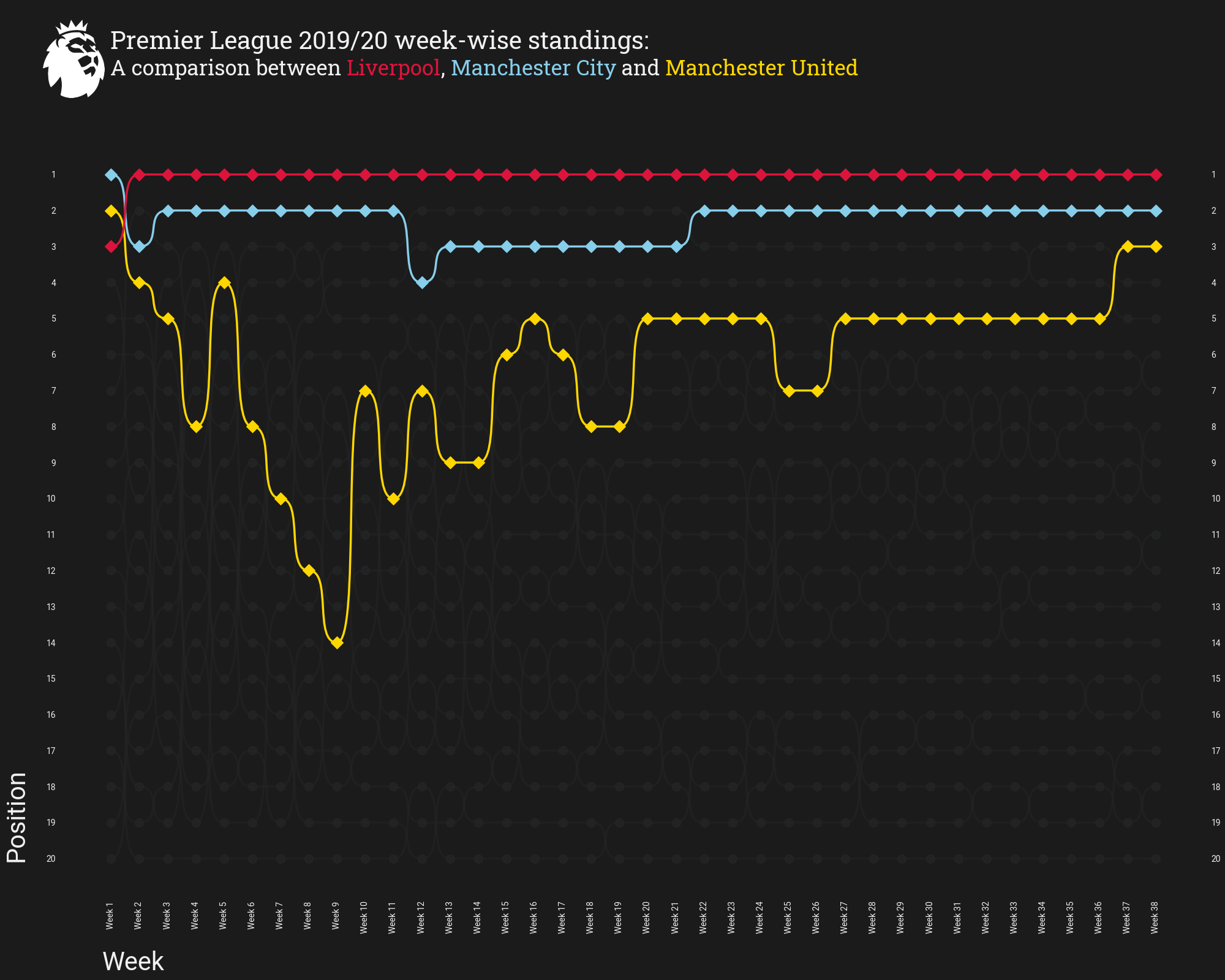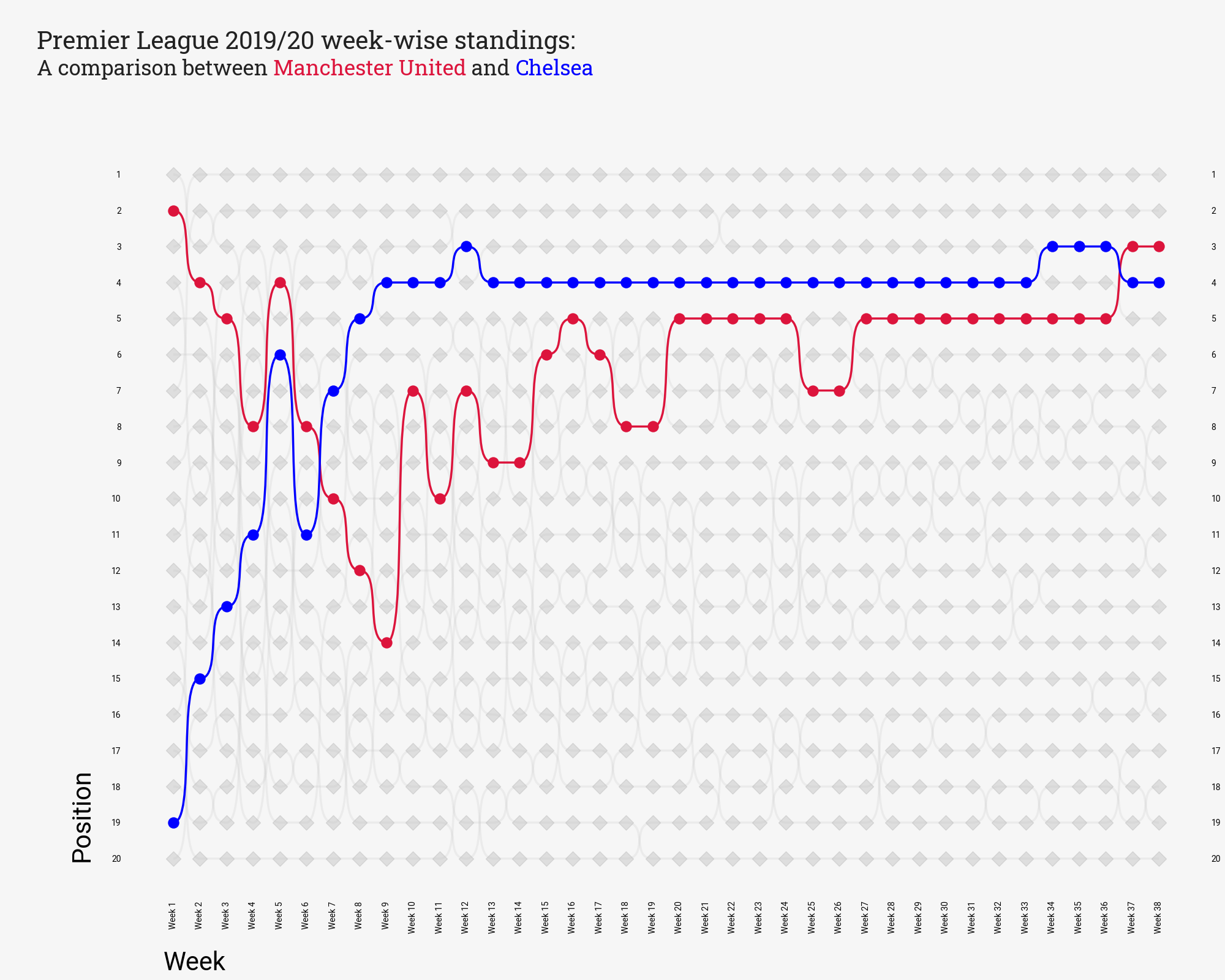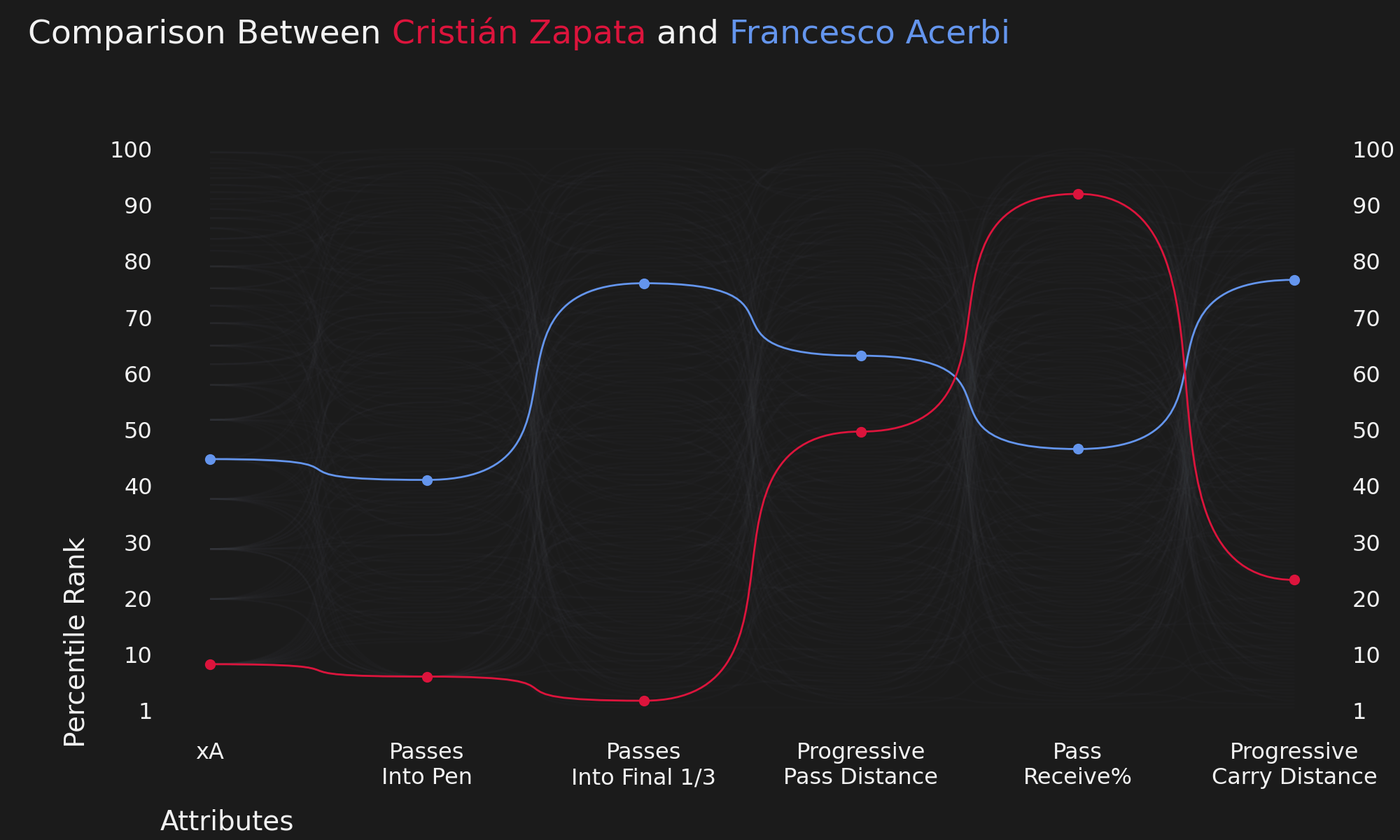# Bumpy Charts¶

• Author: slothfulwave612

• `mplsoccer`, `bumpy_chart` module helps one to plot bumpy charts in a few lines of code.

• Inspired By CJ Mayes

• Here we will show some examples of how to use `mplsoccer` to plot bumpy charts.

```import json
from urllib.request import urlopen

import matplotlib.pyplot as plt
import numpy as np
from PIL import Image
from highlight_text import fig_text

from mplsoccer import Bumpy, FontManager, add_image
```

We will use mplsoccer’s FontManager to load some fonts from Google Fonts. We borrowed the FontManager from the excellent ridge_map library.

```font_normal = FontManager(("https://github.com/google/fonts/blob/main/apache/roboto/"
"static/Roboto-Regular.ttf?raw=true"))
"static/Roboto-Medium.ttf?raw=true"))
```

We will using these images/data in our examples. You can find all the images/data here.

```epl = Image.open(
urlopen("https://github.com/andrewRowlinson/mplsoccer-assets/blob/main/epl.png?raw=true")
)

urlopen("https://github.com/andrewRowlinson/mplsoccer-assets/blob/main/epl.json?raw=true")
)

urlopen(("https://github.com/andrewRowlinson/mplsoccer-assets/blob/main/"
"percentile.json?raw=true"))
)
```

## Making A Bumpy Chart¶

A Bump Chart is a special form of a line plot. This chart is well-suited for exploring changes in rank over time. Using this chart, you can easily compare the position, performance or rankings of multiple observations with respect to each other rather than the actual values itself. We are going to make use of the weekwise standing data for Premier League 2019/20.

```# match-week
match_day = ["Week " + str(num) for num in range(1, 39)]

# highlight dict --> team to highlight and their corresponding colors
highlight_dict = {
"Liverpool": "crimson",
"Man City": "skyblue",
"Man Utd": "gold"
}

# instantiate object
bumpy = Bumpy(
scatter_color="#282A2C", line_color="#252525",  # scatter and line colors
rotate_xticks=90,  # rotate x-ticks by 90 degrees
ticklabel_size=17, label_size=30,  # ticklable and label font-size
scatter_primary='D',  # marker to be used
show_right=True,  # show position on the rightside
plot_labels=True,  # plot the labels
alignment_yvalue=0.1,  # y label alignment
alignment_xvalue=0.065  # x label alignment
)

# plot bumpy chart
fig, ax = bumpy.plot(
x_list=match_day,  # match-day or match-week
y_list=np.linspace(1, 20, 20).astype(int),  # position value from 1 to 20
values=season_dict,  # values having positions for each team
secondary_alpha=0.5,   # alpha value for non-shaded lines/markers
highlight_dict=highlight_dict,  # team to be highlighted with their colors
figsize=(20, 16),  # size of the figure
x_label='Week', y_label='Position',  # label name
ylim=(-0.1, 23),  # y-axis limit
lw=2.5,   # linewidth of the connecting lines
fontproperties=font_normal.prop,   # fontproperties for ticklables/labels
)

# title and subtitle
TITLE = "Premier League 2019/20 week-wise standings:"
SUB_TITLE = "A comparison between <Liverpool>, <Manchester City> and <Manchester United>"

fig.text(0.09, 0.95, TITLE, size=29, color="#F2F2F2", fontproperties=font_bold.prop)

fig_text(
0.09, 0.94, SUB_TITLE, color="#F2F2F2",
highlight_textprops=[{"color": 'crimson'}, {"color": 'skyblue'}, {"color": 'gold'}],
size=25, fig=fig, fontproperties=font_bold.prop
)

epl,
fig,  # figure
0.02, 0.9,  # left and bottom dimensions
0.08, 0.08  # height and width values
)

# if space is left in the plot use this
```## Flip The y-axis¶

If you want to plot positions from the bottom, i.e. the 1st position will be at the bottom and the 20th position will be at the top. You can do it easily. You just have to pass `upside_down=True` inside `plot` function.

```# instantiate object
bumpy = Bumpy(
scatter_color="#282A2C", line_color="#252525",  # scatter and line colors
rotate_xticks=90,  # rotate x-ticks by 90 degrees
ticklabel_size=17, label_size=30,  # ticklable and label font-size
scatter_primary='D',  # marker to be used
show_right=True,  # show position on the rightside
plot_labels=True,  # plot the labels
alignment_yvalue=0.1,  # y label alignment
alignment_xvalue=0.065,  # x label alignment
)

# plot bumpy chart
fig, ax = bumpy.plot(
x_list=match_day,  # match-day or match-week
y_list=np.linspace(1, 20, 20).astype(int),  # position value from 1 to 20
values=season_dict,  # values having positions for each team
secondary_alpha=0.5,   # alpha value for non-shaded lines/markers
highlight_dict=highlight_dict,  # team to be highlighted with their colors
figsize=(20, 16),  # size of the figure
x_label='Week', y_label='Position',  # label name
ylim=(-0.1, 23),  # y-axis limit
lw=2.5,   # linewidth of the connecting lines
upside_down=True,    # <--- to flip the y-axis
fontproperties=font_normal.prop,   # fontproperties for ticklables/labels
)

# title and subtitle
TITLE = "Premier League 2019/20 week-wise standings:"
SUB_TITLE = "A comparison between <Liverpool>, <Manchester City> and <Manchester United>"

fig.text(0.09, 0.95, TITLE, size=29, color="#F2F2F2", fontproperties=font_bold.prop)

fig_text(
0.09, 0.94, SUB_TITLE, color="#F2F2F2",
highlight_textprops=[{"color": 'crimson'}, {"color": 'skyblue'}, {"color": 'gold'}],
size=25, fig=fig, fontproperties=font_bold.prop
)

epl,
fig,  # figure
0.02, 0.9,  # left and bottom dimensions
0.08, 0.08  # height and width values
)

# if space is left in the plot use this
```## Light Theme¶

You can use `background_color`, `scatter_color`, `label_color`, and `line_color` arguments to change the whole theme of the plot. Below is the code demonstrating how to make a light-theme bumpy chart using `mplsoccer`. Below you can also use `scatter_points`, `scatter_primary`, and `scatter_size` arguments inside `Bumpy` method to change markers and their sizes.

```# highlight dict --> team to highlight and their corresponding colors
highlight_dict = {
"Man Utd": "crimson",
"Chelsea": "blue"
}

# instantiate object
bumpy = Bumpy(
background_color="#F6F6F6", scatter_color="#808080",
label_color="#000000", line_color="#C0C0C0",
rotate_xticks=90,  # rotate x-ticks by 90 degrees
ticklabel_size=17, label_size=30,  # ticklable and label font-size
scatter_points='D',   # other markers
scatter_primary='o',  # marker to be used for teams
scatter_size=150,   # size of the marker
show_right=True,  # show position on the rightside
plot_labels=True,  # plot the labels
alignment_yvalue=0.1,  # y label alignment
alignment_xvalue=0.065  # x label alignment
)

# plot bumpy chart
fig, ax = bumpy.plot(
x_list=match_day,  # match-day or match-week
y_list=np.linspace(1, 20, 20).astype(int),  # position value from 1 to 20
values=season_dict,  # values having positions for each team
secondary_alpha=0.2,   # alpha value for non-shaded lines/markers
highlight_dict=highlight_dict,  # team to be highlighted with their colors
figsize=(20, 16),  # size of the figure
x_label='Week', y_label='Position',  # label name
ylim=(-0.1, 23),  # y-axis limit
lw=2.5,   # linewidth of the connecting lines
fontproperties=font_normal.prop,   # fontproperties for ticklabels/labels
)

# title and subtitle
TITLE = "Premier League 2019/20 week-wise standings:"
SUB_TITLE = "A comparison between <Manchester United> and <Chelsea>"

fig.text(0.03, 0.95, TITLE, size=29, color="#222222", fontproperties=font_bold.prop)

fig_text(
0.03, 0.94, SUB_TITLE, color="#222222",
highlight_textprops=[{"color": 'crimson'}, {"color": 'blue'}],
size=25, fig=fig, fontproperties=font_bold.prop
)

# if space is left in the plot use this
```## Player Percentile Rank Viz¶

Here we will create a bumpy-chart that will compare two players based on their percentile rank.

```# attributes and highlight dict
attribute = [
"xA", "Passes\nInto Pen", "Passes\nInto Final 1/3", "Progressive\nPass Distance",
]
highlight_dict = {
"Cristián Zapata": "crimson",
"Francesco Acerbi": "cornflowerblue"
}

# instantiate object
bumpy = Bumpy(
rotate_xticks=0, ticklabel_size=23, label_size=28, scatter="value",
show_right=True, alignment_yvalue=0.15, alignment_xvalue=0.06
)

# plot bumpy chart
fig, ax = bumpy.plot(
x_list=attribute, y_list=np.linspace(1, 100, 11).astype(int), values=player_dict,
secondary_alpha=0.05, highlight_dict=highlight_dict,
figsize=(20, 12),
x_label="Attributes", y_label="Percentile Rank", ylim=(0.5, 12),
upside_down=True,
fontfamily="Liberation Serif"
)

# title and subtitle
TITLE = "Comparison Between <Cristián Zapata> and <Francesco Acerbi>"